## Finding the Volume and Surface Area of Rectangular Solids

### Learning Outcomes

• Find the volume and surface area of rectangular solids

A cheerleading coach is having the squad paint wooden crates with the school colors to stand on at the games. (See the image below). The amount of paint needed to cover the outside of each box is the surface area, a square measure of the total area of all the sides. The amount of space inside the crate is the volume, a cubic measure.

This wooden crate is in the shape of a rectangular solid.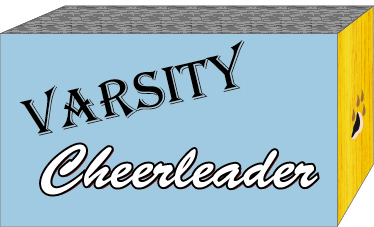Each crate is in the shape of a rectangular solid. Its dimensions are the length, width, and height. The rectangular solid shown in the image below has length $4$ units, width $2$ units, and height $3$ units. Can you tell how many cubic units there are altogether? Let’s look layer by layer.

Breaking a rectangular solid into layers makes it easier to visualize the number of cubic units it contains. This $4$ by $2$ by $3$ rectangular solid has $24$ cubic units.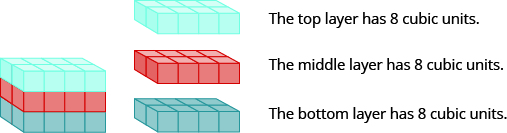Altogether there are $24$ cubic units. Notice that $24$ is the $\text{length}\times \text{width}\times \text{height}\text{.}$The volume, $V$, of any rectangular solid is the product of the length, width, and height.

$V=LWH$

We could also write the formula for volume of a rectangular solid in terms of the area of the base. The area of the base, $B$, is equal to $\text{length}\times \text{width}\text{.}$

$B=L\cdot W$

We can substitute $B$ for $L\cdot W$ in the volume formula to get another form of the volume formula.We now have another version of the volume formula for rectangular solids. Let’s see how this works with the $4\times 2\times 3$ rectangular solid we started with. See the image below.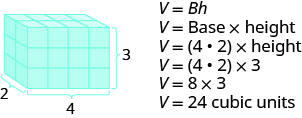To find the surface area of a rectangular solid, think about finding the area of each of its faces. How many faces does the rectangular solid above have? You can see three of them.

$\begin{array}{ccccccc}{A}_{\text{front}}=L\times W\hfill & & & {A}_{\text{side}}=L\times W\hfill & & & {A}_{\text{top}}=L\times W\hfill \\ {A}_{\text{front}}=4\cdot 3\hfill & & & {A}_{\text{side}}=2\cdot 3\hfill & & & {A}_{\text{top}}=4\cdot 2\hfill \\ {A}_{\text{front}}=12\hfill & & & {A}_{\text{side}}=6\hfill & & & {A}_{\text{top}}=8\hfill \end{array}$

Notice for each of the three faces you see, there is an identical opposite face that does not show.

$\begin{array}{l}S=\left(\text{front}+\text{back}\right)\text{+}\left(\text{left side}+\text{right side}\right)+\left(\text{top}+\text{bottom}\right)\\ S=\left(2\cdot \text{front}\right)+\left(\text{2}\cdot \text{left side}\right)+\left(\text{2}\cdot \text{top}\right)\\ S=2\cdot 12+2\cdot 6+2\cdot 8\\ S=24+12+16\\ S=52\text{sq. units}\end{array}$

The surface area $S$ of the rectangular solid shown above is $52$ square units.
In general, to find the surface area of a rectangular solid, remember that each face is a rectangle, so its area is the product of its length and its width (see the image below). Find the area of each face that you see and then multiply each area by two to account for the face on the opposite side.

$S=2LH+2LW+2WH$

For each face of the rectangular solid facing you, there is another face on the opposite side. There are $6$ faces in all.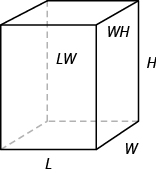### Volume and Surface Area of a Rectangular Solid

For a rectangular solid with length $L$, width $W$, and height $H:$Doing the Manipulative Mathematics activity “Painted Cube” will help you develop a better understanding of volume and surface area.

### example

For a rectangular solid with length $14$ cm, height $17$ cm, and width $9$ cm, find 1. the volume and 2. the surface area.

Solution
Step 1 is the same for both 1. and 2., so we will show it just once.

 Step 1. Read the problem. Draw the figure and label it with the given information.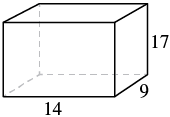1. Step 2. Identify what you are looking for. The volume of the rectangular solid Step 3. Name. Choose a variable to represent it. Let $V$ = volume Step 4. Translate. Write the appropriate formula. Substitute. $V=LWH$ $V=\mathrm{14}cm\cdot 9cm\cdot 17cm$ Step 5. Solve the equation. $V=2,142cm^3$ Step 6. Check We leave it to you to check your calculations. Step 7. Answer the question. The volume is $\text{2,142}$ cubic centimeters.
 2. Step 2. Identify what you are looking for. the surface area of the solid Step 3. Name. Choose a variable to represent it. Let $S$ = surface area Step 4. Translate. Write the appropriate formula. Substitute. $S=2LH+2LW+2WH$ $S=2\left(14cm\cdot 17cm\right)+2\left(14cm\cdot 9cm\right)+2\left(9cm\cdot 17cm\right)$ Step 5. Solve the equation. $S=1,034cm^2$ Step 6. Check: Double-check with a calculator. Step 7. Answer the question. The surface area is $1,034$ square centimeters.

### example

A rectangular crate has a length of $30$ inches, width of $25$ inches, and height of $20$ inches. Find 1. its volume and 2. its surface area.

### Volume and Surface Area of a Cube

A cube is a rectangular solid whose length, width, and height are equal. See Volume and Surface Area of a Cube, below. Substituting, s for the length, width and height into the formulas for volume and surface area of a rectangular solid, we get:

$\begin{array}{ccccc}V=LWH\hfill & & & & S=2LH+2LW+2WH\hfill \\ V=s\cdot s\cdot s\hfill & & & & S=2s\cdot s+2s\cdot s+2s\cdot s\hfill \\ V={s}^{3}\hfill & & & & S=2{s}^{2}+2{s}^{2}+2{s}^{2}\hfill \\ & & & & S=6{s}^{2}\hfill \end{array}$

So for a cube, the formulas for volume and surface area are $V={s}^{3}$ and $S=6{s}^{2}$.

### Volume and Surface Area of a Cube

For any cube with sides of length $s$,### example

A cube is $2.5$ inches on each side. Find 1. its volume and 2. its surface area.

Solution
Step 1 is the same for both 1. and 2., so we will show it just once.

 Step 1. Read the problem. Draw the figure and label it with the given information.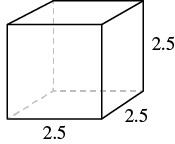1. Step 2. Identify what you are looking for. The volume of the cube Step 3. Name. Choose a variable to represent it. Let V = volume Step 4. Translate. Write the appropriate formula. $V={s}^{3}$ Step 5. Solve. Substitute and solve. $V={\left(2.5in\right)}^{3}$ $V=15.625in^3$ Step 6. Check: Check your work. Step 7. Answer the question. The volume is $15.625$ cubic inches.
 2. Step 2. Identify what you are looking for. The surface area of the cube Step 3. Name. Choose a variable to represent it. Let S = surface area Step 4. Translate. Write the appropriate formula. $S=6{s}^{2}$ Step 5. Solve. Substitute and solve. $S=6\cdot {\left(2.5in\right)}^{2}$ $S=37.5in^2$ Step 6. Check: The check is left to you. Step 7. Answer the question. The surface area is $37.5$ square inches.

### example

A notepad cube measures $2$ inches on each side. Find 1. its volume and 2. its surface area.

## Contribute!

Did you have an idea for improving this content? We’d love your input.